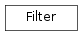# 4.3. `pyopus.optimizer.optfilter` — Filter-based point acceptance¶Filter support for constrained optimization (PyOPUS subsystem name: FILT)

class `pyopus.optimizer.optfilter.``Filter`(hmax=0.0, debug=0)

All points are stored in a dictionary with keys of the form (f,h).

The value stored alongside (f,h) can be anything.

A point (f,h) dominates (f0,h0) if

• h<h0 and f<=f0 or
• h<=h0 and f<f0

(f,h) is dominated by the filter if h>hmax.

No point in the filter dominates any other point in the filter.

hmax=0 results in extreme barrier behavior.

`accept`(f, h, misc)

Checks a point against the filter.

If h>hmax the point does not dominate nor is dominated. Such a point is rejected.

If filter is empty, a point is accepted. Such a point is considered to dominate filter.

If point dominates any filter point it is accepted. Dominated filter points are deleted.

If a point is dominated by any filter point it is rejected. If a point does not dominate nor is dominated it is accepted.

Returns boolean tuple (dominates, dominated, accepted).

`bestFeasible`()

Returns (f,h,misc) of the feasible point.

Returns (None, None, None) if no such point exists.

static `dominates`(f, h, f0, h0)

Returns `True` if (f,h) dominates (f0,h0).

`leastInfeasible`()

Returns (f,h,misc) of the infeasible point with lowest h.

Returns (None, None, None) if no such point exists.

`mostInfeasible`()

Returns (f,h,misc) of the infeasible point with highest h.

Returns (None, None, None) if no such point exists.

`orderedHlist`()

Returns h values in increasing order.

`orderedPoints`()

Returns the f and h values ordered by increasing h.

`position`(f, h)

Returns the position of h in the ordered list of h values.

0 ... feasible point 1 ... best infeasible point 2 ... second infeasible point ...

Returns `None` if the point is not in the filter.

`reset`(hmax=None)

Resets the filter.

Points are stored in a dictionary with h as key. Dictionary values are tuples of the form (f, misc). There can be only one point for every h0 value.

`updateHmax`(hmax)

Updates hmax and purges points with h>hmax.